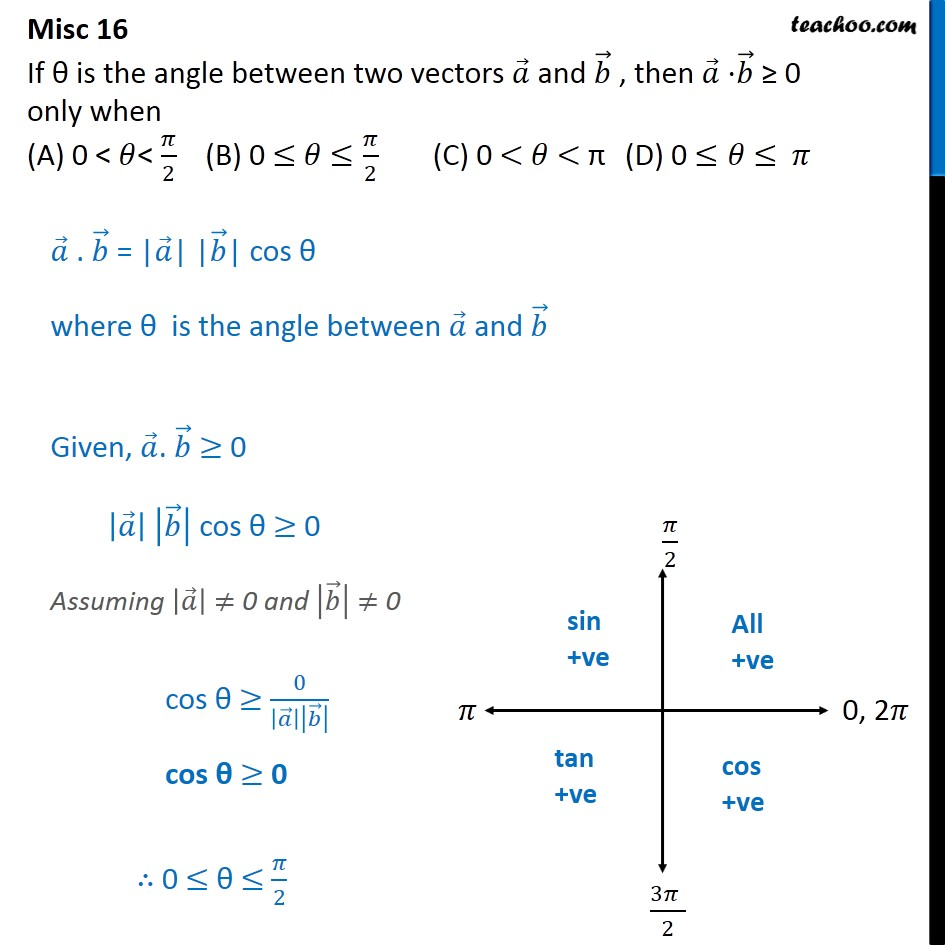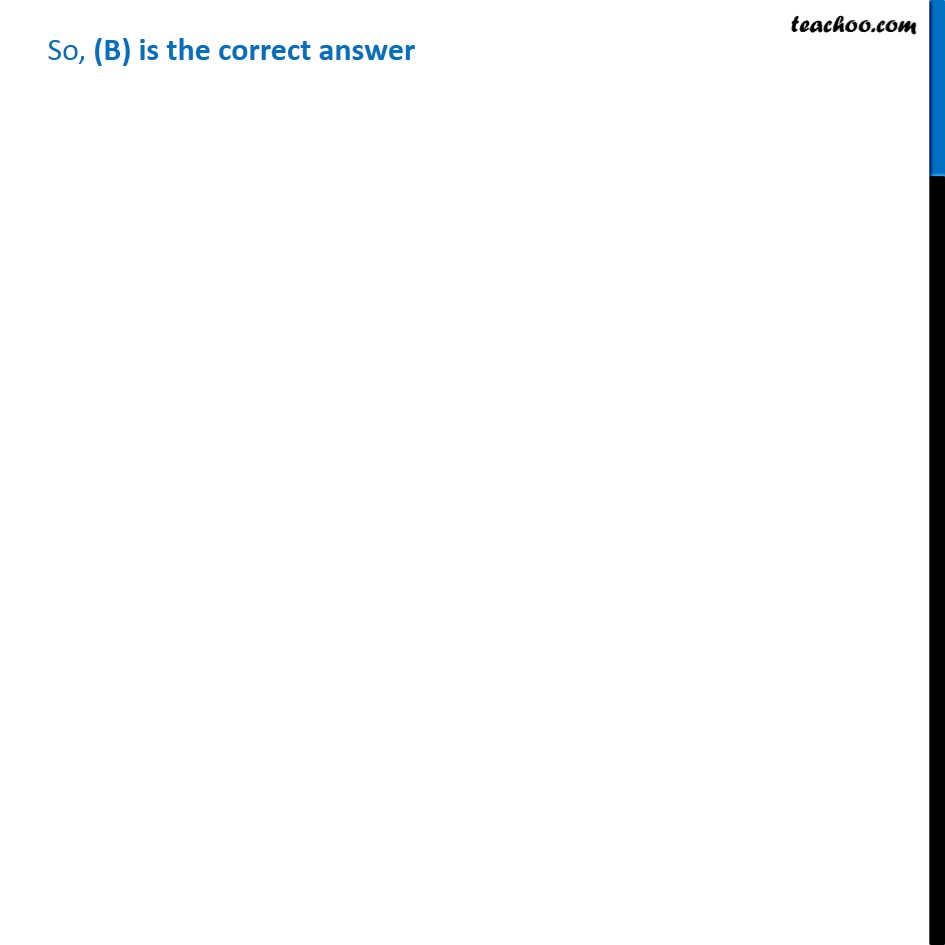1. Chapter 10 Class 12 Vector Algebra
2. Serial order wise
3. Miscellaneous

Transcript

Misc 16 If is the angle between two vectors and , then 0 only when (A) 0 < < 2 (B) 0 2 (C) 0 < < (D) 0 . = | | | | cos where is the angle between and Given, . 0 cos 0 Assuming 0 and 0 cos 0 cos 0 0 2 So, (B) is the correct answer

Miscellaneous